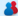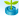Hi GuestHomePageJobsWalkInArticles
 ----- Select Country ----- ----- Select Location ----- ----- Select Category -----

:) Latest Topic
 AJAX VB.NET SQL Query UDDI CLASS JAVASCRIPT SharePoint Interview SilverLight WCF general knowledge
ASP.NET
 :) Hot Jobs

 :) Latest Articles

 Write Roman number from 1 to 10000 count Question Posted on 08 Jun 2019 Home >> Gernal Knowledge >> general knowledge >> Write Roman number from 1 to 10000 count

 Write Roman number from 1 to 10000 count Below is the list of most common numbers comes in between 1 to 10000 1=I 2=II 3=III 4=IV 5=V 6=VI 7=VII 8=VIII 9=IX 10=X 11=XI 12=XII 13=XIII 14=XIV 15=XV 16=XVI 17=XVII 18=XVIII 19=XIX 20=XX 30=XXX 40=XL 50=L 60=LX 70=LXX 80=LXXX 90=XC 100=C 200=CC 300=CCC 400=CD 500=D 600=DC 700=DCC 800=DCCC 900=CM 1000=M 2000=MM 3000=MMM 4000=M(V) 5000=(V) 10000=(X)

 Other Important Questions GK and Current affairs june 2015 part 1 Basic General Knowledge for improve skill Basic General Knowledge Questions Write Roman number from 1 to 10000 count 2019 G20 Osaka summit Countries List